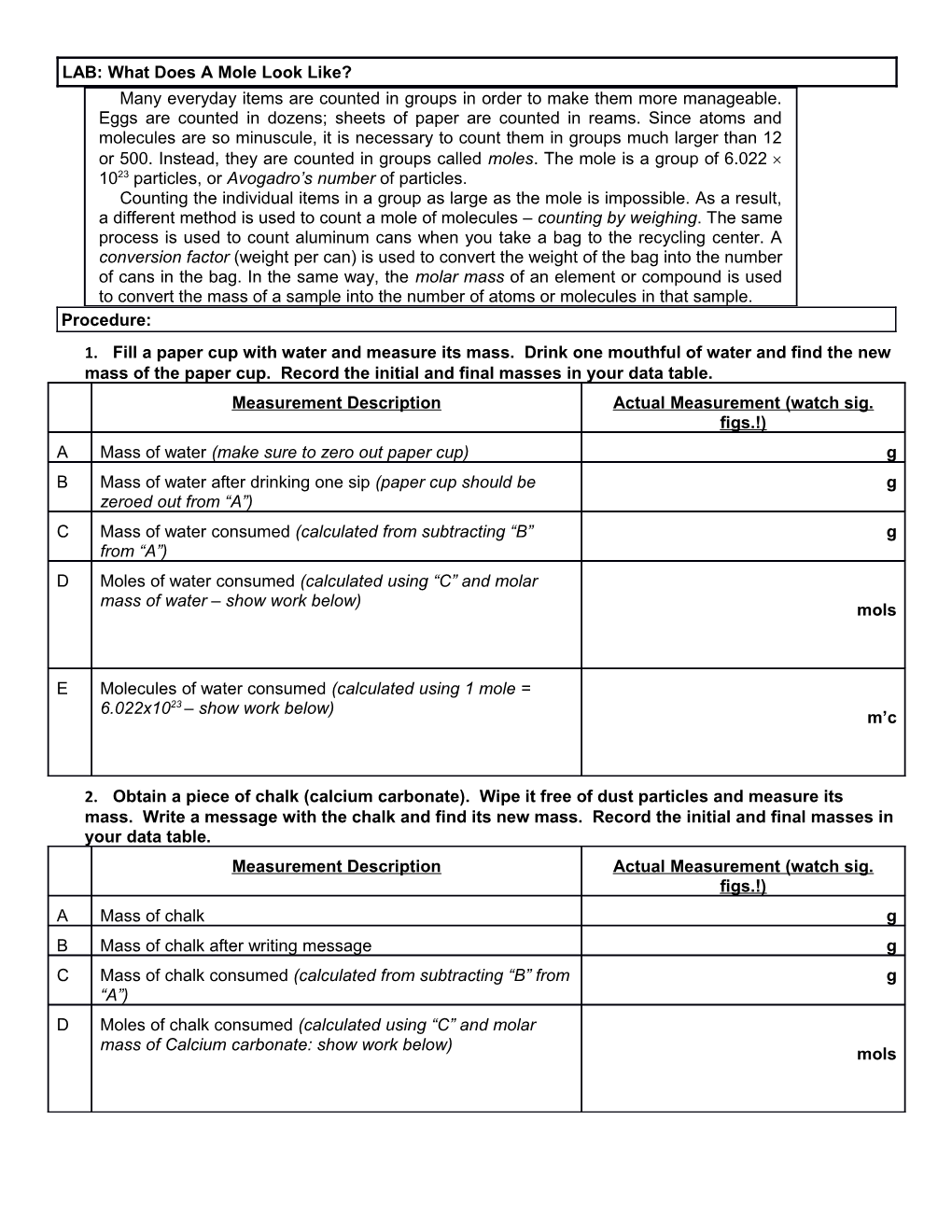# LAB: What Does a Mole Look Like?LAB: What Does A Mole Look Like?

Many everyday items are counted in groups in order to make them more manageable. Eggs are counted in dozens; sheets of paper are counted in reams. Since atoms and molecules are so minuscule, it is necessary to count them in groups much larger than 12 or 500. Instead, they are counted in groups called moles. The mole is a group of 6.022 1023 particles, or Avogadro’s number of particles.
Counting the individual items in a group as large as the mole is impossible. As a result, a different method is used to count a mole of molecules – counting by weighing. The same process is used to count aluminum cans when you take a bag to the recycling center. A conversion factor (weight per can) is used to convert the weight of the bag into the number of cans in the bag. In the same way, the molar mass of an element or compound is used to convert the mass of a sample into the number of atoms or molecules in that sample.

Procedure:

1. Fill a paper cup with water and measure its mass. Drink one mouthful of water and find the new mass of the paper cup. Record the initial and final masses in your data table.

Measurement Description / Actual Measurement (watch sig. figs.!)
A / Mass of water (make sure to zero out paper cup) / g
B / Mass of water after drinking one sip (paper cup should be zeroed out from “A”) / g
C / Mass of water consumed (calculated from subtracting “B” from “A”) / g
D / Moles of water consumed (calculated using “C” and molar mass of water – show work below) / mols
E / Molecules of water consumed (calculated using 1 mole = 6.022x1023 – show work below) / m’c
1. Obtain a piece of chalk (calcium carbonate). Wipe it free of dust particles and measure its mass. Write a message with the chalk and find its new mass. Record the initial and final masses in your data table.

Measurement Description / Actual Measurement (watch sig. figs.!)
A / Mass of chalk / g
B / Mass of chalk after writing message / g
C / Mass of chalk consumed (calculated from subtracting “B” from “A”) / g
D / Moles of chalk consumed (calculated using “C” and molar mass of Calcium carbonate: show work below) / mols
1. Obtain a piece of Aluminum foil from the teacher. Measure and record the length and width of the square to the nearest 0.1 cm. Find the mass of the foil. Return the foil to the teacher. The density of Al is 2.70g/cm3.

Measurement Description / Actual Measurement (watch sig. figs.!)
A / Length of Al foil
Width of Al foil / cm
cm
B / Density of Al / 2.70 g/cm3
C / Mass of Al foil / g
D / Volume of Al foil (calculated using Density and mass – show work below) / cm3
E / Moles in Al foil (hint: molar mass – show work below) / mols
F / Atoms in Al foil (hint: 1 mole = 6.02x1023 atoms – show work below) / atoms

Analysis Questions – Answer in complete sentences!!

1. Identify the conversion factors you used and specify how you used them.
1. If you consume 5 grams each of water, chalk, and Aluminum, will you consume more water molecules, more chalk molecules, or more Aluminum atoms? Explain/Show Work.
1. If you consume 5 moles each of water, chalk, and Aluminum, will you consume more water molecules, more chalk molecules, or more Aluminum atoms? Explain/Show Work.